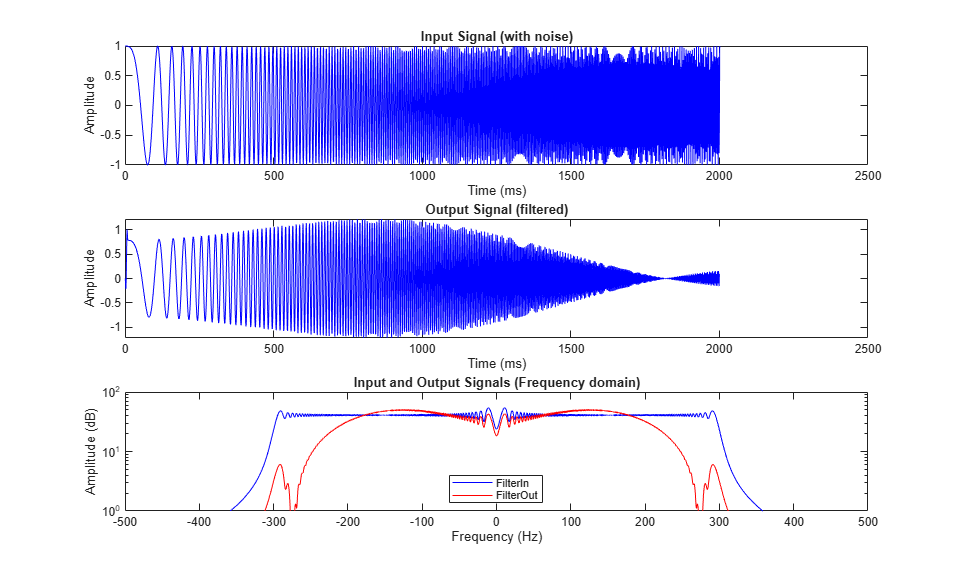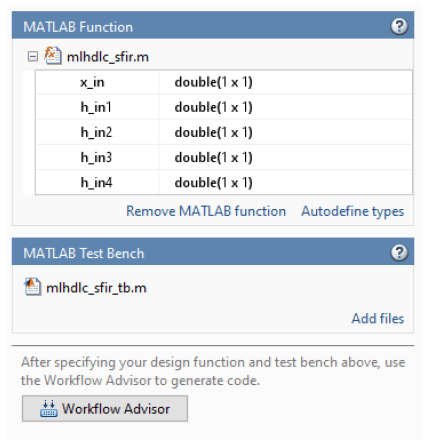# Basic HDL Code Generation and FPGA Synthesis from MATLAB

This example shows how to create a HDL Coder™ project, generate code for your MATLAB® design, and synthesize the HDL code. In this example, you:

1. Create a MATLAB HDL Coder project.

2. Add the design and test bench files to the project.

3. Start the HDL Workflow Advisor for the MATLAB design.

4. Run fixed-point conversion and HDL code generation.

5. Generate a HDL test bench from the MATLAB test bench.

6. Verify the generated HDL code by using a HDL simulator. This example uses ModelSim® as the tool.

7. Synthesize the generated HDL code by using a synthesis tool. This example uses Xilinx® Vivado® as the tool.

### FIR Filter MATLAB Design

The MATLAB design `mlhdlc_sfir` is a simple symmetric FIR filter.

```design_name = 'mlhdlc_sfir'; testbench_name = 'mlhdlc_sfir_tb'; ```

Review the MATLAB design.

```open(design_name); ```
```%%%%%%%%%%%%%%%%%%%%%%%%%%%%%%%%%%%%%%%%%%%%%%%%%%%%%%%%%%%%%%%%%%%%%%%%%% % MATLAB design: Symmetric FIR Filter % % Introduction: % % We can reduce the complexity of the FIR filter by leveraging its symmetry. % Symmetry for an n-tap filter implies, coefficient h0 = coefficient hn-1, % coefficient, h1 = coefficient hn-2, etc. In this case, the number of % multipliers can be approximately halved. The key is to add the % two data values that need to be multiplied with the same coefficient % prior to performing the multiplication. % % Key Design pattern covered in this example: % (1) Filter states represented using the persistent variables % (2) Filter coefficients passed in as parameters % Copyright 2011-2019 The MathWorks, Inc. %#codegen function [y_out, delayed_xout] = mlhdlc_sfir(x_in,h_in1,h_in2,h_in3,h_in4) % Symmetric FIR Filter % declare and initialize the delay registers persistent ud1 ud2 ud3 ud4 ud5 ud6 ud7 ud8; if isempty(ud1) ud1 = 0; ud2 = 0; ud3 = 0; ud4 = 0; ud5 = 0; ud6 = 0; ud7 = 0; ud8 = 0; end % access the previous value of states/registers a1 = ud1 + ud8; a2 = ud2 + ud7; a3 = ud3 + ud6; a4 = ud4 + ud5; % multiplier chain m1 = h_in1 * a1; m2 = h_in2 * a2; m3 = h_in3 * a3; m4 = h_in4 * a4; % adder chain a5 = m1 + m2; a6 = m3 + m4; % filtered output y_out = a5 + a6; % delayout input signal delayed_xout = ud8; % update the delay line ud8 = ud7; ud7 = ud6; ud6 = ud5; ud5 = ud4; ud4 = ud3; ud3 = ud2; ud2 = ud1; ud1 = x_in; end ```

### FIR Filter MATLAB Test Bench

A MATLAB testbench `mlhdlc_sfir_tb` exercises the filter design by using a representative input range. Review the MATLAB test bench `mlhdlc_sfir_tb`.

```open(testbench_name); ```
```%%%%%%%%%%%%%%%%%%%%%%%%%%%%%%%%%%%%%%%%%%%%%%%%%%%%%%%%%%%%%%%%%%%%%%%%%% % MATLAB test bench for the FIR filter %%%%%%%%%%%%%%%%%%%%%%%%%%%%%%%%%%%%%%%%%%%%%%%%%%%%%%%%%%%%%%%%%%%%%%%%%% % Copyright 2011-2019 The MathWorks, Inc. clear mlhdlc_sfir; T = 2; dt = 0.001; N = T/dt+1; sample_time = 0:dt:T; df = 1/dt; sample_freq = linspace(-1/2,1/2,N).*df; % input signal with noise x_in = cos(2.*pi.*(sample_time).*(1+(sample_time).*75)).'; % filter coefficients h1 = -0.1339; h2 = -0.0838; h3 = 0.2026; h4 = 0.4064; len = length(x_in); y_out = zeros(1,len); x_out = zeros(1,len); for ii=1:len data = x_in(ii); % call to the design 'mlhdlc_sfir' that is targeted for hardware [y_out(ii), x_out(ii)] = mlhdlc_sfir(data, h1, h2, h3, h4); end figure('Name', [mfilename, '_plot']); subplot(3,1,1); plot(1:len,x_in,'-b'); xlabel('Time (ms)') ylabel('Amplitude') title('Input Signal (with noise)') subplot(3,1,2); plot(1:len,y_out,'-b'); xlabel('Time (ms)') ylabel('Amplitude') title('Output Signal (filtered)') freq_fft = @(x) abs(fftshift(fft(x))); subplot(3,1,3); semilogy(sample_freq,freq_fft(x_in),'-b'); hold on semilogy(sample_freq,freq_fft(y_out),'-r') hold off xlabel('Frequency (Hz)') ylabel('Amplitude (dB)') title('Input and Output Signals (Frequency domain)') legend({'FilterIn', 'FilterOut'}, 'Location','South') axis([-500 500 1 100]) ```

### Test the Original MATLAB Algorithm

To avoid run-time errors, simulate the design by using the test bench.

```mlhdlc_sfir_tb ```### Set Up HDL Simulator and Synthesis Tool Path

If you want to synthesize the generated HDL code, before you use HDL Coder to generate code, set up your synthesis tool path. To set up the path to your synthesis tool, use the hdlsetuptoolpath function. For example, if your synthesis tool is Xilinx Vivado:

```hdlsetuptoolpath('ToolName','Xilinx Vivado','ToolPath',... 'C:\Xilinx\Vivado\2020.2\bin\vivado.bat'); ```

```!vivado ```

If you want to simulate the generated HDL code by using a HDL test bench, you can use an HDL simulator such as ModelSim®. You must have already installed the HDL simulator.

### Create an HDL Coder Project

To create an HDL Coder project:

1. Create a project by running this command:

```coder -hdlcoder -new sfir_project ```

2. For MATLAB Function, add the MATLAB design `mlhdlc_sfir`. Add `mlhdlc_sfir_tb.m` as the MATLAB test bench.

3. Click Autodefine types and use the recommended types for the MATLAB design. The code generator infers data types by running the test bench.### Create Fixed-Point Versions of Algorithm and Test Bench

1. Click the Workflow Advisor button to open the Workflow Advisor. You see that the Define Input Types task has passed.

2. Run the Fixed-Point Conversion task. The Fixed-Point Conversion tool opens in the right pane.

When you run fixed-point conversion, to propose fraction lengths for floating-point data types, HDL Coder uses the Default word length. In this tutorial, the Default word length is `14`. The advisor provides a default Safety Margin for Simulation Min/Max of `0%`. The advisor adjusts the range of the data by this safety factor. For example, a value of `4` specifies that you want a range of at least `4` percent larger. See also Floating-Point to Fixed-Point Conversion.

### Select Code Generation Options and Generate HDL Code

Before you generate HDL code, if you want to deploy the code onto a target platform, specify the synthesis tool. In the Code Generation Target task, leave Workflow to `Generic ASIC/FPGA` and specify `Xilinx Vivado` as the Synthesis Tool. If you don't see the synthesis tool, click Refresh list. Run this task.

In the HDL Code Generation task, by using the tabs on the right side of this task, you can specify additional code generation options.

1. By default, HDL Coder generates VHDL® code. To generate Verilog or SystemVerilog code, in the Target tab, choose `Verilog` or `SystemVerilog` as the Language.

2. To generate a code generation report with comments and traceability links, in the Coding style tab, select Include MATLAB source code as comments and Generate report.

3. To optimize your design, you can use the distributed pipelining optimization. In the Optimizations tab, specify `1` for Input pipelining and Output pipelining and then select Distribute pipeline registers. To learn more, see Distributed Pipelining.

4. Click Run to generate Verilog or SystemVerilog code.

Examine the log window and click the links to explore the generated code and the reports.

### Generate HDL Test Bench and Simulate the Generated Code

HDL Coder generates a HDL test bench, runs the HDL test bench by using a HDL simulator, and verifies whether the HDL simulation matches the numerics and latency of the fixed-point MATLAB simulation.

To generate a HDL test bench and simulate the generated code, in the HDL Verification > Verify with HDL Test Bench task:

1. In the Output Settings tab, select Generate HDL test bench.

2. To simulate the generated test bench, set the Simulation Tool to `ModelSim`. You must have already installed ModelSim.

3. To specify generation of HDL test bench code and test bench data in separate files, in the Test Bench Options tab, select Multi-file test bench.

4. Click the Run button.

The task generates an HDL test bench, then simulates the fixed-point design by using the selected simulation tool, and generates a compilation report and a simulation report.

### Synthesize Generated HDL Code

HDL Coder synthesizes the HDL code on the target platform and generates area and timing reports for your design based on the target device that you specify.

To synthesize the generated HDL code:

1. Run the Create project task.

This task creates a Xilinx Vivado synthesis project for the HDL code. HDL Coder uses this project in the next task to synthesize the design.

2. Select and run the Run Synthesis task.

This task launches the synthesis tool in the background, opens the synthesis project, compiles the HDL code, synthesizes the design, and generates netlists and area and timing reports.

3. Select and run the Run Implementation task.

This task launches the synthesis tool in the background, runs place and route on the design, and generates pre- and post-route timing information for use in critical path analysis and back annotation of your source model.